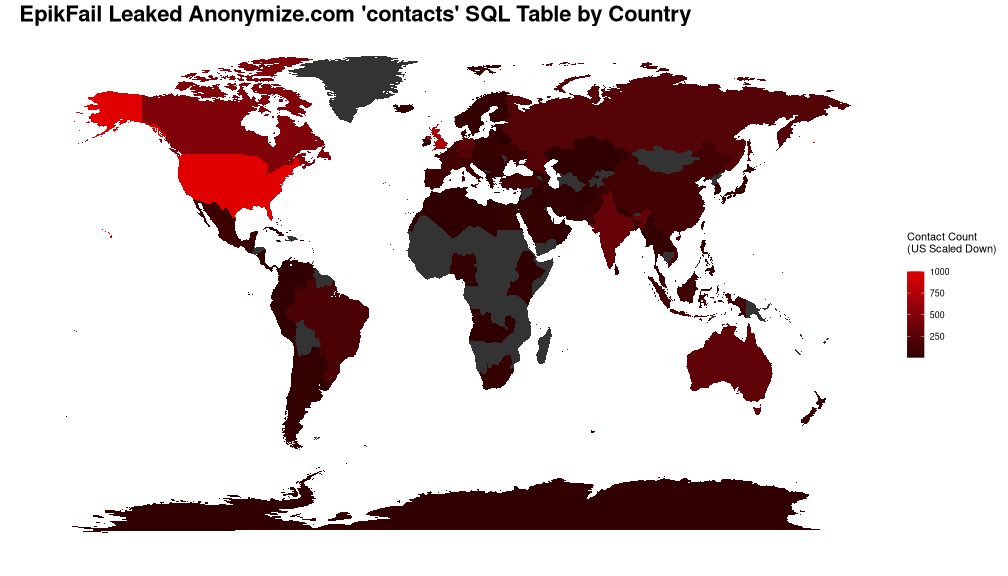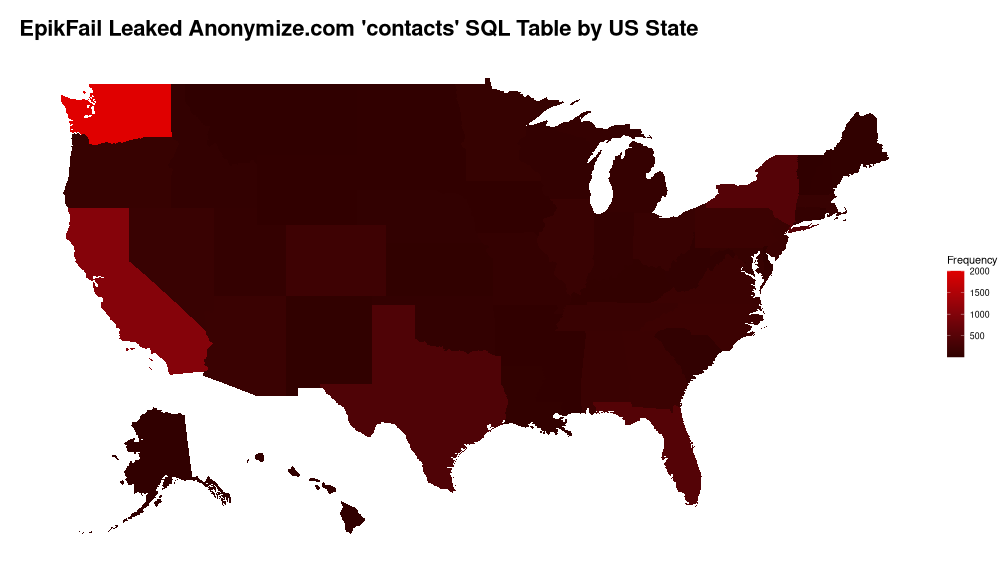#39;contactsBrad Cable#39; SQL Table Maps

# EpikFail Leaked Anonymize.com 'contacts' SQL Table Maps

https://therecord.media/anonymous-hacks-and-leaks-data-from-domain-registrar-epik/

The EpikFail breach has a contacts database table for Anonymize.com that can be extracted with country codes to map via the following.

#!/bin/bash

zcat EpikFail/sql/epik_anonymize.sql.gz | \
grep -A18 "CREATE TABLE .contacts" | \
tail -n 18 | \
sed -r "s/^[\t ]+.([^ ]+). .*$/\1/g" | \ tr "\n" "," | \ sed -r "s/,$/\n/g" > contacts.csv

zcat EpikFail/sql/epik_anonymize.sql.gz | \
grep -E "INSERT INTO .contacts" | \
sed -r "s/\),$$/\n/g" | \ sed -r "s/^INSERT INTO .* VALUES \(//g" | \ sed -r "s/$$;$//g" | \ sed "s/\\\'//g" >> contacts.csv  ## Load Data Into R library(ggplot2) library(mapproj)  ## Loading required package: maps  library(scales) library(fiftystater)  source("shared/country_code_cleanup.R") source("shared/world_mapper.R")  state_codes <- read.csv("shared/state_codes.csv")  contacts <- read.csv("contacts.csv", sep=",", quote="'")  contacts[contacts$country == "NOT AVAILABLE" | contacts$country == "n/a" | contacts$country == 'e' | contacts$country == 'o',] <- NA contacts$country[contacts$country == "0000"] <- "US" contacts$country[contacts$country == "Italia"] <- "IT" contacts$country[contacts$country == "KOREA"] <- "KR" contacts$country[contacts$country == "Niedersachsen"] <- "DE"  contact_countries <- country_code_cleanup(contacts$country)
write.csv(contact_countries, "contact_countries.csv", quote=FALSE, row.names=FALSE)


USA has 19803 entries, which scales too high… scaling down for visibility.

contact_countries$Count[contact_countries$Country == "USA"] <- 1000

contact_states <- contacts$state[contacts$country == "US" & contacts$state != "" & contacts$state != "*" & contacts$state != "NULL" & contacts$state != "Not Applicable" & contacts$state != "Private" & contacts$state != "USVI" & contacts$state != "Grand Cayman"] contact_states <- tolower(contact_states) contact_states[contact_states == "california"] <- "ca" contact_states[contact_states == "florida"] <- "fl" contact_states[contact_states == "illinois"] <- "il" contact_states[contact_states == "kansas"] <- "ks" contact_states[contact_states == "maryland"] <- "md" contact_states[contact_states == "nevada"] <- "nv" contact_states[contact_states == "new hampshire"] <- "nh" contact_states[contact_states == "new jersey"] <- "nj" contact_states[contact_states == "new york"] <- "ny" contact_states[contact_states == "north carolina"] <- "nc" contact_states[contact_states == "texas"] <- "tx" contact_states[contact_states == "washington"] <- "wa" contact_states <- substr(contact_states, 0, 2)  contact_states <- as.data.frame(table(contact_states)) names(contact_states) <- c("State.Code", "Frequency") write.csv(contact_states, "contact_states.csv", quote=FALSE, row.names=FALSE)  Washington State has 13756 entries, scaling this down as well. contact_states$Frequency[contact_states$State.Code == "wa"] <- 2000  contact_states <- merge(contact_states, state_codes)  ## World Map g <- world_mapper(contact_countries) g <- g + labs( title="EpikFail Leaked Anonymize.com 'contacts' SQL Table by Country", fill="Contact Count\n(US Scaled Down)\n", x="", y="" ) g <- g + scale_fill_continuous(low="#300000", high="#E00000", guide="colorbar") g <- g + theme(plot.title = element_text(face="bold", size=22)) g## US States Map g <- ggplot(contact_states, aes(map_id=State.Name)) g <- g + ggtitle("EpikFail Leaked Anonymize.com 'contacts' SQL Table by US State") g <- g + geom_map(aes(fill=Frequency), map=fifty_states) g <- g + expand_limits(x=fifty_states$long, y=fifty_states\$lat)
g <- g + scale_x_continuous(breaks=NULL) + scale_y_continuous(breaks=NULL)
g <- g + scale_fill_continuous(low="#300000", high="#E00000", guide="colorbar")
g <- g + xlab("") + ylab("") + theme(
panel.background=element_blank(),
plot.title = element_text(
face="bold", size=22,
margin=margin(0.5, 0.5, 0.5, 0.5, "cm"), debug=FALSE
),
)
g